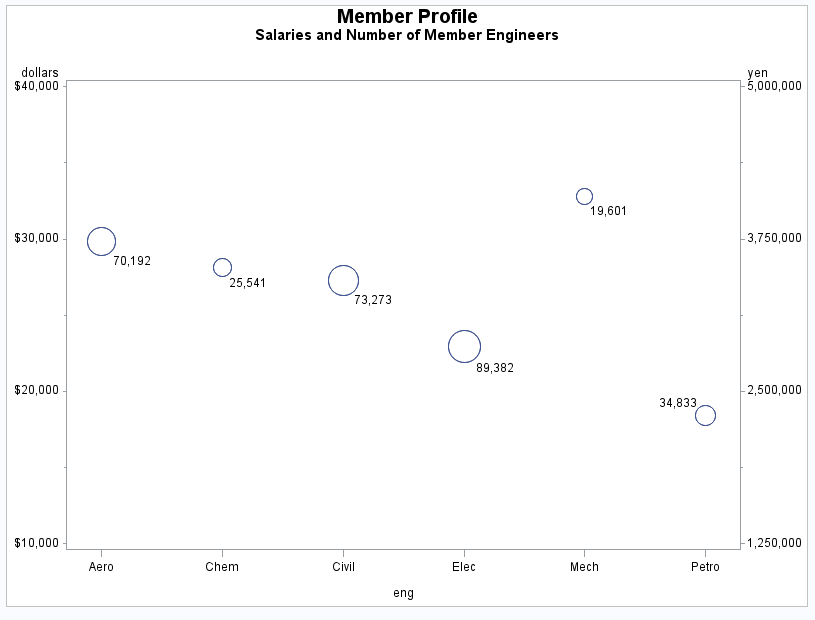# Example 3: Adding a Right Vertical Axis

 Features: BUBBLE statement options : VAXIS= HAXIS= HMINOR= VMINOR= BLABEL Other features: AXIS statement FORMAT statement GOPTIONS statement option: BORDER Sample library member: GPLAXIS1
This example modifies Labeling and Sizing Plot Bubbles to show how a BUBBLE2 statement generates a right vertical axis that displays the values of the vertical coordinates in a different scale from the scale that is used for the left vertical axis. Salary values are scaled by dollars on the left vertical axis and by yen on the right vertical axis.
BUBBLE and BUBBLE2 statement options control the appearance of the graph. In particular, the VAXIS options calibrate the axes so that the data points are identical and only one set of bubbles appears.
Note: If the data points are not identical, two sets of bubbles are displayed.## Program

`goptions reset=all border;`
```data jobs;
length eng \$5;
input eng dollars num;
datalines;
Civil 27308 73273
Aero  29844 70192
Elec  22920 89382
Mech  32816 19601
Chem  28116 25541
Petro 18444 34833
;```
```data jobs2;
set jobs;
yen=dollars*125;
run;```
```title1 "Member Profile";
title2 "Salaries and Number of Member Engineers";```
`axis1 offset=(5,5);`
```proc gplot data=jobs2;
format dollars dollar7. num yen comma9.0;
bubble dollars*eng=num / haxis=axis1
vaxis=10000 to 40000 by 10000
hminor=0
vminor=1
blabel;

bubble2 yen*eng=num / vaxis=1250000 to 5000000 by 1250000
vminor=1;
run;
quit;```

## Program Description

Set the graphics environment.
`goptions reset=all border;`
Create the JOBS data set. The data set JOBS contains average salary data for several categories of engineer. It also indicates the number of engineers in each category.
```data jobs;
length eng \$5;
input eng dollars num;
datalines;
Civil 27308 73273
Aero  29844 70192
Elec  22920 89382
Mech  32816 19601
Chem  28116 25541
Petro 18444 34833
;```
Create the data set JOBS2 and calculate variable YEN. The DATA step uses a SET statement to read the JOBS data set.
```data jobs2;
set jobs;
yen=dollars*125;
run;```
Define titles.
```title1 "Member Profile";
title2 "Salaries and Number of Member Engineers";```
Define horizontal-axis characteristics.
`axis1 offset=(5,5);`
Generate bubble plot with second vertical axis. In the BUBBLE statement, the HAXIS= option specifies the AXIS1 definition and the VAXIS= option scales the left axis. In the BUBBLE2 statement, the VAXIS= option scales the right axis. Both axes represent the same range of monetary values. The BUBBLE and BUBBLE2 statements ensure that the bubbles generated by each statement are identical by coordinating specifications on any options in these statements.
```proc gplot data=jobs2;
format dollars dollar7. num yen comma9.0;
bubble dollars*eng=num / haxis=axis1
vaxis=10000 to 40000 by 10000
hminor=0
vminor=1
blabel;

bubble2 yen*eng=num / vaxis=1250000 to 5000000 by 1250000
vminor=1;
run;
quit;```